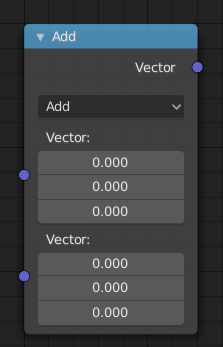# Vector Math Node¶Vector Math Node.

The Vector Math node performs the selected math operation on the input vectors.

## Inputs¶

The inputs of the node are dynamic. Some inputs are only available in certain operations. For instance, the Scale input is only available in the Scale operator.

Vector

Input vector A.

Vector

Input vector B.

Scale

Input Scale.

## Properties¶

Operation

The vector math operator to be applied on the input vectors.

The sum of A and B.

Subtract

The difference between A and B.

Multiply

The entrywise product of A and B. $$(A.x * B.x, A.y * B.y, A.z * B.z)$$

Divide

The entrywise division of A by B. Division by zero returns zero. $$(A.x / B.x, A.y / B.y, A.z / B.z)$$

Cross Product

The cross product of A and B.

Project

The projection of A onto B.

Reflect

The reflection of A around the normal B. B need not be normalized.

Dot Product

The dot product of A and B.

Distance

The distance between A and B.

Length

The length of A.

Scale

The result of multiplying A by the scalar input Scale.

Normalize

The result of normalizing A.

Wrap

Wrap.

Snap

The result of rounding A to the largest integer multiple of B less than or equal A.

Floor

The entrywise floor of A.

Ceil

The entrywise ceiling of A.

Modulo

The entrywise modulo of A by B.

Fraction

The fractional part of A.

Absolute

The entrywise absolute value of A.

Minimum

The entrywise minimum from A and B.

Maximum

The entrywise maximum from A and B.

Sine

The Sine of A.

Cosine

The Cosine of A.

Tangent

The Tangent of A.

## Outputs¶

The output of the node is dynamic. It is either a vector or a scalar depending on the operator. For instance, the Length operator has a scalar output while the Add operator has a vector output.

Vector

Output vector.

Value

Output value.